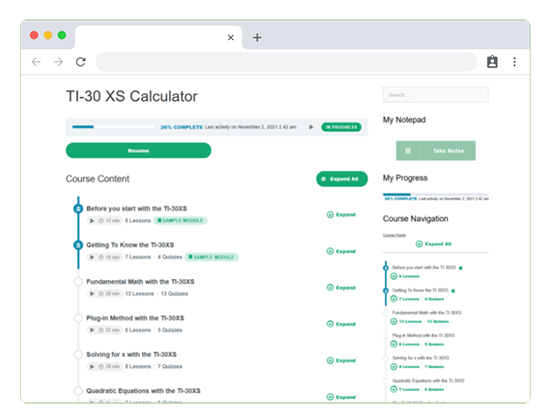# TI-30 XS Calculator Course

The TI-30 XS Calculator Course teaches you how to use this scientific calculator to solve GED questions related to math. These types of questions appear on the GED® Math, Science, and Social Studies tests.

## What you’ll learn

• Understand the basic and advanced functions of the TI-30 XS calculator
• Understand which calculator functions are needed for a given problem
• Apply knowledge of calculator functions to a range of mathematical calculationsThis course includes 7 modules with lessons that cover everything related to utilizing this scientific calculator on 3 subjects of the GED Test.

This course shows you how to solve many different types of mathematical problems using the TI-30 XS calculator, from fractions and proportions to advanced equations and inequalities.

When you take the Onsego course, you will be gradually exposed to techniques, leveraging your skills to the next level.

Every lesson contains a video, text, and a short quiz, and this combination gives you the possibility of getting familiar with the topics in multiple ways.

At the end of each module, a more extended practice test with explanations helps you summarize what you have learned.

## FAQ

How long does it take to complete the TI-30 XS calculator course?

This course includes 4 learning hours of materials. So, if you study just 1 hour a day, you will complete this course within a week.

Can I take this course instead of Math?

The TI-30 XS course teaches you how to answer mathematical calculation questions, however, translating words to algebra (word problems) topics are not part of this course. If you are not familiar with these topics we suggest combining the calculator course with the Math Essentials. Both courses are included in the My Advantage plan.

Can I use a different calculator?

No, you need to use the TI-30XS calculator. On the GED test, you are allowed to use ONLY this type of calculator. You can buy this calculator at every Walmart, BestBuy shop, or Amazon website. It costs around \$15.We also introduce you to apps and other free resources. On the GED test, there’s also an embedded, on-screen version.

Can I check how it works?

Sure, here is a sample lesson.

### Sample Lesson

Exponents. On the test, there are always questions that include exponents.

So, let’s check how to solve these types of problems.

To calculate exponents, we will need to use the caret sign.

This sign looks like this

As you see, It’s a primary key.Let’s calculate how much is 8 to the power of three.

Option A: 512, or Option B: 164. Press 8, and then choose a caret sign.You will see a small square next to 8. Now, press three. The small square changes to 3.Our screen displays 8 to the power of three. So, press enter to check the result. It’s 512.Option A is correct.